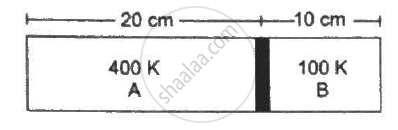Department of Pre-University Education, KarnatakaPUC Karnataka Science Class 11
Advertisement Remove all ads

# Figure Shows a Cylindrical Tube of Length 30 Cm Which is Partitioned by a Tight-fitting Separator. the Separator is Very Weakly Conducting and Can Freely Slide Along the Tube. Ideal Gases Are Filled - Physics

Sum

Figure shows a cylindrical tube of length 30 cm which is partitioned by a tight-fitting separator. The separator is very weakly conducting and can freely slide along the tube. Ideal gases are filled in the two parts of the vessel. In the beginning, the temperatures in the parts A and B are 400 K and 100 K respectively. The separator slides to a momentary equilibrium position shown in the figure. Find the final equilibrium position of the separator, reached after a long time.Advertisement Remove all ads

#### Solution

Let the initial pressure of the chambers A and B be PA1 and PB1 , respectively .

Let the final pressure of chambers A and B be PA2 and PB2 , respectively .

Let the CSA be A.

VA1 = 0.20 A

TA1 = 400 K

VB1 = 0.1 A

TB1 = 100 K

At first equilibrium , both side pressures will be the same .

⇒ PA1 = PB1

Let the final temperature at equilibrium be T .then ,

(P_(A1)V_(A1))/(T_(A1)) =( P_(A2)V_(A2))/T

⇒ (P_(A1)0.2A)/400 = (P_(A2)V_(A2))/T

⇒ P_(A2 )=( P_(A1)0.2AT)/(400V_(A2)) ......(1)

For Second chamber :

(P_(B1)V_(B1))/(T_(B1)) = (P_(B2)V_(B2))/T

⇒ (P_(B1)0.1A)/100 = (P_(B2)V_(B2))/T

⇒ P_(B2) = (P_(B1)0.1AT)/(100V_(B2))....(2)

At Second equilibrium , pressures on both sides will be the same .

⇒ P_(A2) = P_(B2)

⇒ (P_(A1)0.2AT)/(400V_(A2)) = (P_(B1)0.1AT)/(100V_(B2))

⇒ P_(A1)/(2V_(A2)) = P_(B1)/V_(B2)

⇒ V_(B2) = 2V_(A2)  ...........(3)

Now ,

V_(B2) + V_(A2) = 0.3A

⇒ 3V_(A2) = 0.3A

⇒ V_(A2) = 0.1A

Let V_(A2) be  lA.

⇒ l = 0.1 m

⇒ l = 10 cm

Concept: Kinetic Theory of Gases- Assumptions
Is there an error in this question or solution?
Advertisement Remove all ads

#### APPEARS IN

HC Verma Class 11, Class 12 Concepts of Physics Vol. 2
Chapter 2 Kinetic Theory of Gases
Q 36 | Page 36
Advertisement Remove all ads

#### Video TutorialsVIEW ALL 

Advertisement Remove all ads
Share
Notifications

View all notifications

Forgot password?